# Algebraic Data Types: Exponents

Episode #9 • Mar 26, 2018 • Subscriber-Only

We continue our explorations into algebra and the Swift type system. We show that exponents correspond to functions in Swift, and that by using the properties of exponents we can better understand what makes some functions more complex than others.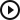Algebraic Data Types: Exponents
Introduction
00:05
A correction
01:05
Exponents
04:50
Functions
05:30
Property #1
08:58
Property #2
12:32
Property #3
14:57
The algebra of in-out
22:41
The algebra of throws
24:28
What’s the point?
28:07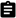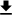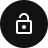### Introduction

In the last episode on algebraic data types we started to get a glimpse of what it means to see algebraic structure in the Swift type system. We saw that forming structs corresponds to a kind of multiplication of the types inside the struct. Then we saw that forming enums corresponded to a kind of summation of all the types on the inside. And finally we used this intuition to figure how to properly model a datatype so that impossible states are not representable, and enforced by the compiler.

In this episode we are we are going to look at the next piece of algebra that is not captured by just plain sums and products: exponentiation. We will see that it helps build our intuition for how function arrows act with respect to other constructions, and even allow us to understand what makes a function more or less complicated.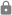This episode is for subscribers only.

### Subscribe to Point-Free

Access this episode, plus all past and future episodes when you become a subscriber.

See plans and pricing

### Exercises

1. Prove the equivalence of `1^a = 1` as types. This requires re-expressing this algebraic equation as types, and then defining functions between the types that are inverses of each other.

2. What is `0^a`? Prove an equivalence. You will need to consider `a = 0` and `a != 0` separately.

3. How do you think generics fit into algebraic data types? We’ve seen a bit of this with thinking of `Optional<A>` as `A + 1 = A + Void`.

4. Show that sets with values in `A` can be represented as `2^A`. Note that `A` does not require any `Hashable` constraints like the Swift standard library `Set<A>` requires.

5. Define `intersection` and `union` functions for the above definition of set.

6. How can dictionaries with keys in `K` and values in `V` be represented algebraically?

7. Implement the following equivalence:

``````func to<A, B, C>(_ f: @escaping (Either<B, C>) -> A) -> ((B) -> A, (C) -> A) {
fatalError()
}

func from<A, B, C>(_ f: ((B) -> A, (C) -> A)) -> (Either<B, C>) -> A {
fatalError()
}
``````
8. Implement the following equivalence:

``````func to<A, B, C>(_ f: @escaping (C) -> (A, B)) -> ((C) -> A, (C) -> B) {
fatalError()
}

func from<A, B, C>(_ f: ((C) -> A, (C) -> B)) -> (C) -> (A, B) {
fatalError()
}
``````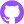0009-algebraic-data-types-pt-2